Home Introduction About Us kelvinprobe.com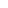Figures A to C (below) show various electron energy diagrams for two conducting specimens, 1 and 2.Figure A
Electron energy level diagram for two conducting specimens, where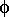1 and2 are the work functions of the materials, and1 and2 represent their fermi levels.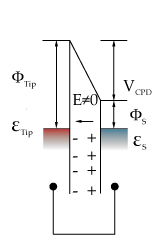Figure B
If an external electrical contact is made between the two electrodes their fermi levels equalise and the resulting flow of charge (in direction indicated) produces a potential gradient, termed the contact potential Vc, between the plates. The two surfaces become equally and oppositely charged.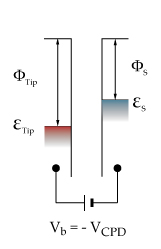Figure C
Inclusion of a variable "backing potential" Vb in the external circuit, permits biasing of one electrode with respect to the other. At the unique point where the (average) electric field between the plates vanishes, resulting in a null output signal.

#### Theory Behind the Kelvin Probe

The renowned Scottish physicist Sir William Thomson, later Lord Kelvin showed over a century ago that a potential is generated between the surfaces of two conductors when they are brought into electrical contact. This contact potential depends on the work functions of the materials being used. The work function is the amount of energy needed to release electrons from a material's surface, and is related to the material's optical, electrical and mechanical properties. When two materials with different work functions are brought together, electrons in the material with the lower work function flow to the one with the higher work function. If these materials are made into a parallel plate capacitor, equal and opposite surface charges form. The voltage developed over this capacitor is called the contact potential and measuring it is done by applying an external backing potential to the capacitor until the surface charges disappear, at that point the backing potential will equal the contact potential.
The wf difference between two surfaces can be found by measuring the flow of charge when the two conducting materials are connected (Figure B). However, this produces a once only measurement, as the surfaces become charged, and the charge must dissipate before another measurement can be made. By using a vibrating probe, a varying capacitance is produced. This is given by: C=Q/V=(epsilon.A)/t (Equation 1) Where C is the capacitance, Q is the Charge, V is the Potential, Epsilon 0 is the permittivity of the dielectric (in an air probe the dielectric is air). A is the surface area of the capacitor and d is the separation between the plates. Therefore, as the separation d increases the capacitance C decreases. As the charge remains constant, the voltage V must increase.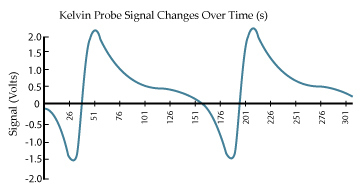As the probe oscillates above the sample the voltage change is recorded. The peak-to-peak output voltage Vptp, is given by: Vptp = (Delta V - Vb)R.C0.w.e.sin(w.t + f) (Equation 2) Where Delta V represents the voltage difference between probe tip and sample, Vb is the externally applied emf used to balance (or null) the circuit, R is the I/V converter feedback resistance, C0 is the mean Kelvin Probe capacitance, Omega the angular frequency of vibration and Theta the phase angle. Epsilon is the modulation index (d1/d0) where d0 is the average distance between the sample and the probe tip and d1 is the amplitude of oscillation of the probe.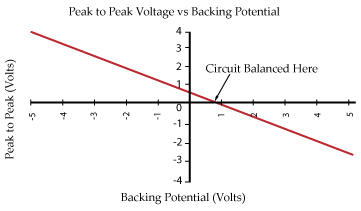The backing potential Vb is set to a range of potentials and a plot of Vptp verses Vb is made. As can be seen from Equation 2 this is a straight line. When Vptp = 0 the Work Function or Contact Potential of the surface is equal and opposite to Vb. Traditional phase sensitive detection methods utilise a LIA (lock in amplifier) to detect the null output condition. However, these systems have the disadvantage that at the balanced point, the signal to noise (S/N) ratio reaches a minimum, and noise creates an offset voltage. A better method is to set Vb to a range of voltages and then extrapolate to the null signal condition, thus working to a high S/N ratio.Single Electron Transistor Device results scan
Kelvin Probe 50micron tip measuring a Single Electron Transistor Device
Scanning Kelvin Probe
UHV Kelvin Probe
Solar Panels
Scanning Kelvin Probe